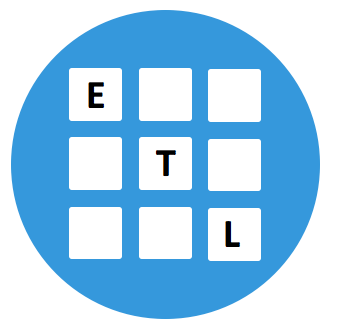# Expressions Template Library (ETL)Realization
• Baptiste Wicht
Keywords
• High Performance
• Matrix/Vector operations
• Matrix Multiplication
• Convolution
• High-Performance
• C++
Our skills
High-Performance
Matrix computations

## Matrix/Vector computations library for C++

ETL is a header only library for C++ that provides vector and matrix classes with support for Expression Templates to perform very efficient operations on them.

At this time, the library support compile-time sized matrix and vector and runtime-sized matrix and vector with all element-wise operations implemented. It also supports multiple forms of 1D and 2D convolution, matrix multiplication (several algorithms) and 1D and 2D FFT. Most of the kernels are highly optimized with vector operations and parallelized. It can also be accelerated using BLAS libraries.

## Features

• Unary and binary operations on matrix/vector (C = A * 1.01 + (B * D) / 2.0 – E)
• Matrix multiplications
• Convolutions
• Fast Fourier Transform
• Q/R and L/U decomposition
• Pooling and upsampling

The DLL library for machine learning is based on the ETL library for all optimized operations.